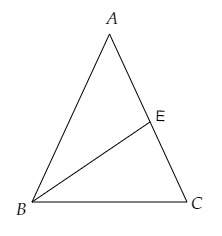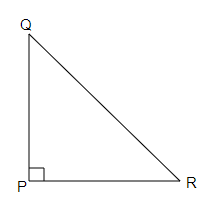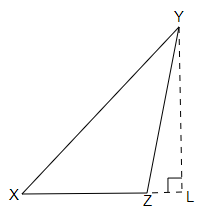# Draw rough sketches for the following:$(a)$ In √¢ÀÜ‚ÄÝABC, BE is a median.$(b)$ In √¢ÀÜ‚ÄÝPQR, PQ and PR are altitudes of the triangle.$(c)$ In √¢ÀÜ‚ÄÝXYZ, YL is an altitude in the exterior of the triangle.

To do:

We have to draw rough sketches for the following:

(a) In $\triangle ABC, BE$ is a median.

(b) In $\triangle PQR, PQ$ and $PR$ are altitudes of the triangle.

(c) In $\triangle XYZ, YL$ is an altitude in the exterior of the triangle.

Solution :

(a)

median of a triangle is a line segment joining a vertex to the mid-point of the side opposite side.In $\triangle \mathrm{ABC}, \mathrm{BE}$ is the median.

(b) An altitude of a triangle is the perpendicular drawn from the vertex of the triangle to the opposite side.$\Delta PQR$ is a right-angled triangle in which $PQ$ and $QR$ are altitudes.

(c)$\Delta XYZ$ is an obtuse-angled triangle $YL$ is an altitude in the exterior of the triangle.